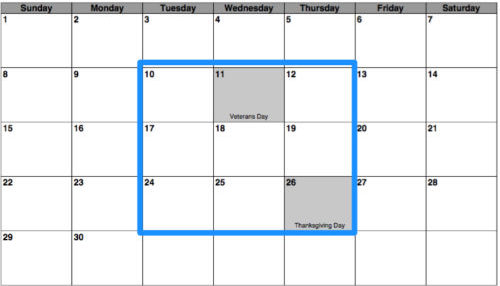Home Free Lessons The Calendar Problem• Author:
• Posted:
• Topics:
,
• Type:
• Keywords:

#### Overview

Materials: Calendar, Hundreds chart, pencil and paper, calculator (optional)
Common Core: 4.OA.1, 4.OA.3, 4.OA.5, 4.NBT.5, MP1, MP2, MP3, MP6, MP7, MP8

How can you add up these numbers so quickly?

#### Why we love this lesson

Simple to launch, instantly engaging and accessible, this puzzle is a beautiful demonstration of the usefulness of multiplication. Not only that, figuring out why the trick works, even when you notice it does, requires exactly the kind of thinking we want our students to do.

#### The Launch

Draw a 3 by 3 square on a calendar (only getting days from a single month inside the square).Challenge your students to add up the numbers as quick as they can. You can even let them use calculators. But, no matter how fast they add, you can always beat them. What’s the fast way to add these nine numbers together?

#### The Work

Once the students understand the question, let them work in pairs to try to figure it out. The teacher can circulate through the classroom, asking students to explain their ideas, and, as necessary, providing hints to students who are having trouble finding traction with the problem.

Hint 1.
Just trying a bunch of examples and looking for patterns, either by listing or by coloring them in on a hundreds chart, can help a lot, especially if you organize the outcomes. If we try different squares on the November page above, we’ll get numbers like:
99 108 117

Shifting the square to the right seems to add 9.
Will this always work? Why would it? What would happen for different months?

Hint 2
There may be a way to see something if you use a 2 by 2 square instead of a 3 by 3 square.
Or you could try a 1 by 3 rectangle.

Hint 3
Maybe there’s a way to break the addition problem down into manageable pieces.
What if you added each row of the 3 by 3 square up, then added those sums? Or what if you added the columns first?

#### The Wrap

Bring students together to share their solutions. There are many ways to answer this question, and hopefully students will come up with several. One possible solution and argument is below.

The answer turns out always to be 9 times the number at the center of the square. This is because every opposite pair of numbers averages out to the number in the center. For example, instead of adding 10 and 12, we could just add two 11s. Instead of adding 3 and 19, we could just add two 11s. Adding all nine numbers comes out to the same as adding nine of the middle number, which is just a multiplication problem. That’s how the teacher is able to do it so easily!

#### Extensions

• Is there a quick way to add up the numbers in a 4 by 4 square?